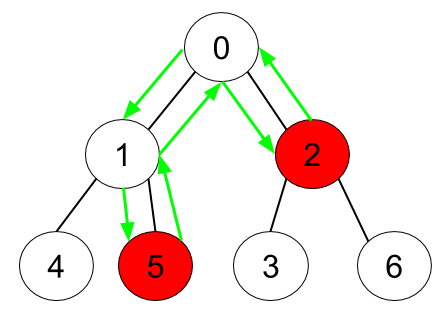All contents and pictures on this website come from the Internet and are updated regularly every week. They are for personal study and research only, and should not be used for commercial purposes. Thank you for your cooperation.

# 1443. Minimum Time to Collect All Apples in a Tree

Given an undirected tree consisting of `n` vertices numbered from 0 to `n-1`, which has some apples in their vertices. You spend 1 second to walk over one edge of the tree. Return the minimum time in seconds you have to spend in order to collect all apples in the tree starting at vertex 0 and coming back to this vertex.

The edges of the undirected tree are given in the array `edges`, where `edges[i] = [fromi, toi]` means that exists an edge connecting the vertices `fromi` and `toi`. Additionally, there is a boolean array `hasApple`, where `hasApple[i] = true` means that vertex `i` has an apple, otherwise, it does not have any apple.

Example 1:```Input: n = 7, edges = [[0,1],[0,2],[1,4],[1,5],[2,3],[2,6]], hasApple = [false,false,true,false,true,true,false]
Output: 8
Explanation: The figure above represents the given tree where red vertices have an apple. One optimal path to collect all apples is shown by the green arrows.
```

Example 2:```Input: n = 7, edges = [[0,1],[0,2],[1,4],[1,5],[2,3],[2,6]], hasApple = [false,false,true,false,false,true,false]
Output: 6
Explanation: The figure above represents the given tree where red vertices have an apple. One optimal path to collect all apples is shown by the green arrows.
```

Example 3:

```Input: n = 7, edges = [[0,1],[0,2],[1,4],[1,5],[2,3],[2,6]], hasApple = [false,false,false,false,false,false,false]
Output: 0
```

Constraints:

• `1 <= n <= 10^5`
• `edges.length == n-1`
• `edges[i].length == 2`
• `0 <= fromi, toi <= n-1`
• `fromi < toi`
• `hasApple.length == n`

Medium

Normal